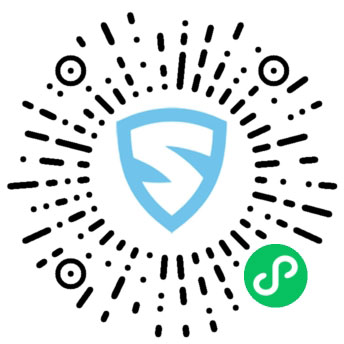# 在yii2应用中，使用imagine库生成分享图实战imagine图片库
二维码生成库
一个好看的字体
一张海报（自行用PS处理）

imagine

imagine 支持三种底层的图像处理库（GD、Imagick和Gmagick），GD是最老的图片库，自然处理能力也不如另外两种，本次我使用Imagick作为底层支持，关于PHP如何安装Imagick扩展可参考 https://www.cnblogs.com/shanhubei/p/16969236.html

`composer require imagine/imagine`

qrcode-library的安装也非常简单，依然是composer。

`composer require 2amigos/qrcode-library`

```use Imagine\Imagick\Imagine;

public function actionShare(\$id){
\$imagine = new Imagine();
//VarDumper::dump(\$image,10,true);
}```

imagine和image对象都正常输出了。

文本内容是什么
用什么字体
字号大小
字体颜色
从哪个坐标开始

注：imagine和笛卡尔坐标系不同，详情见 文档

`Yii::getAlias('@app')."/fonts/yahei.ttf";`

```use Imagine\Imagick\Imagine;
use Imagine\Image\Palette\RGB;
use Imagine\Image\Point;

public function actionShare(\$id){
\$imagine = new Imagine();

\$palette = new RGB();
\$color = \$palette->color("000000");

\$point = new Point(0,0);

\$font = \$imagine->font(Yii::getAlias('@app')."/fonts/yahei.ttf",12,\$color);

\$image->draw()->text("请使用PHP循环出本周一到本周日",\$font,\$point);
\$image->show('jpg');
}```

`text(string \$string, AbstractFont \$font, PointInterface \$position, int \$angle = 0, int \$width = null)`

```use Imagine\Image\Palette\RGB;
use Imagine\Image\Point;```

```\$palette = new RGB();
\$color = \$palette->color("000000");```

`\$font = \$imagine->font(Yii::getAlias('@app')."/fonts/yahei.ttf",12,\$color);`

`\$point = new Point(0,0);`

字体大小
坐标位置

```\$point = new Point(44,230);
\$font = \$imagine->font(Yii::getAlias('@app')."/fonts/yahei.ttf",24,\$color);```

```\$font = \$imagine->font(Yii::getAlias('@app')."/fonts/yahei.ttf",24,\$color);
\$text = \$font->wrapText("请使用PHP循环出本周一到本周日",400);```

wrapText方法的第二个参数代表文本最大长度，超过了即为换行，然后将wrapText作用后的\$text再传给 image->draw()->text(); 即可。

```// vendor/imagine/imagine/src/Image/FontInterface.php:59
public function wrapText(\$string, \$maxWidth, \$angle = 0){
\$words = explode(' ', \$string);
foreach (\$words as \$word) {
if (\$currentLine === null) {
\$currentLine = \$word;
} else {
\$testLine = \$currentLine . ' ' . \$word;
\$testbox = \$this->box(\$testLine, \$angle);
if (\$testbox->getWidth() <= \$maxWidth) {
\$currentLine = \$testLine;
} else {
\$lines[] = \$currentLine;
\$currentLine = \$word;
}
}
}
.....
return implode("\n", \$lines);
}```

**那对中文怎么办？**没关系，从官方wrapText的方法我们可以改造出子的方法，只不过每个词的话不不再是空格，我进行了如下改造。

```\$lines = array();
\$maxWidth = 420;
\$currentLine = null;
\$text = "请使用PHP循环出本周一到本周日";
for(\$i = 0;\$i < mb_strlen(\$text,'UTF-8');\$i++){
\$word = mb_substr(\$text,\$i,1,'UTF-8');
if (\$currentLine === null) {
\$currentLine = \$word;
} else {
\$testLine = \$currentLine.\$word;
\$testbox = \$font->box(\$testLine, 0);
if (\$testbox->getWidth() <= \$maxWidth) {
\$currentLine = \$testLine;
} else {
\$lines[] = \$currentLine;
\$currentLine = \$word;
}
}
}
if (\$currentLine !== null) {
\$lines[] = \$currentLine;
}

\$text = implode("\n", \$lines);```

```\$height = \$font->box(\$model->title)->getHeight();// 获得字的高度。\$model->title 就是输出的内容
\$lines = array();
\$currentLine = null;
for(\$i = 0;\$i < mb_strlen(\$model->title,'UTF-8');\$i++){
\$word = mb_substr(\$model->title,\$i,1,'UTF-8');
if (\$currentLine === null) {
\$currentLine = \$word;
} else {
\$testLine = \$currentLine.\$word;
\$testbox = \$font->box(\$testLine, 0);
if (\$testbox->getWidth() <= 420) {
\$currentLine = \$testLine;
} else {
\$lines[] = \$currentLine;
\$currentLine = \$word;
}
}
}
if (\$currentLine !== null) {
\$lines[] = \$currentLine;
}

// 获得lines数组
foreach(\$lines as \$key=>\$value){
\$point = new Point(40,(\$key == 0 ? 230 : (230 + (\$height + 10)*\$key)));
\$image->draw()->text(\$value,\$font,\$point,0);
}

\$image->show('jpg');```

```use Imagine\Image\Point\Center;

\$dateText = date('Y-m-d',\$model->publish_date);
\$dateFont = \$imagine->font(Yii::getAlias('@app')."/fonts/yahei.ttf",12,\$color);
\$dateBox = \$dateFont->box(\$dateText);
\$dateCenterPosition = new Center(\$dateBox);
\$image->draw()->text(\$dateText,\$dateFont,new Point((\$image->getSize()->getWidth()/2 - \$dateCenterPosition->getX()),420));```

生成二维码
写入二维码到分享图

```use Da\QrCode\QrCode;

\$qrCode->writeFile(\$path);```

##写入二维码到分享图

```\$water = \$imagine->open(\$path);
\$image->paste(\$water,new Point(150,520));```### 觉得文章有用就打赏一下文章作者

#### 支付宝扫一扫打赏#### 微信扫一扫打赏